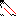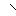# MINLPLib

### A Library of Mixed-Integer and Continuous Nonlinear Programming Instances

#### Instance: ex9_2_3

 Formatsⓘ ams gms lp mod nl osil pip Primal Boundsⓘ 5.00000000 p1 ( gdx sol ) (infeas: 7e-15)-0.00000000 p2 ( gdx sol ) (infeas: 0) Dual Boundsⓘ -0.00000000 (ANTIGONE)-0.00000000 (BARON)0.00000000 (COUENNE)-0.00000000 (LINDO)0.00000000 (SCIP) Referencesⓘ Floudas, C A, Pardalos, Panos M, Adjiman, C S, Esposito, W R, Gumus, Zeynep H, Harding, S T, Klepeis, John L, Meyer, Clifford A, and Schweiger, C A, Handbook of Test Problems in Local and Global Optimization, Kluwer Academic Publishers, 1999.Visweswaran, V, Floudas, C A, Ierapetritou, M G, and Pistikopoulos, E N, A Decomposition-Based Global Optimization Approach for Solving Bilevel Linear and Quadratic Programs. Chapter 10 in Floudas, C A and Pardalos, P M, Eds, State of the Art in Global Optimization, Kluwer Academic Publishers, 1996, 139-162. Sourceⓘ Test Problem ex9.2.3 of Chapter 9 of Floudas e.a. handbook Added to libraryⓘ 31 Jul 2001 Problem typeⓘ QCP #Variablesⓘ 16 #Binary Variablesⓘ 0 #Integer Variablesⓘ 0 #Nonlinear Variablesⓘ 12 #Nonlinear Binary Variablesⓘ 0 #Nonlinear Integer Variablesⓘ 0 Objective Senseⓘ min Objective typeⓘ linear Objective curvatureⓘ linear #Nonzeros in Objectiveⓘ 4 #Nonlinear Nonzeros in Objectiveⓘ 0 #Constraintsⓘ 15 #Linear Constraintsⓘ 9 #Quadratic Constraintsⓘ 6 #Polynomial Constraintsⓘ 0 #Signomial Constraintsⓘ 0 #General Nonlinear Constraintsⓘ 0 Operands in Gen. Nonlin. Functionsⓘ Constraints curvatureⓘ indefinite #Nonzeros in Jacobianⓘ 40 #Nonlinear Nonzeros in Jacobianⓘ 12 #Nonzeros in (Upper-Left) Hessian of Lagrangianⓘ 12 #Nonzeros in Diagonal of Hessian of Lagrangianⓘ 0 #Blocks in Hessian of Lagrangianⓘ 6 Minimal blocksize in Hessian of Lagrangianⓘ 2 Maximal blocksize in Hessian of Lagrangianⓘ 2 Average blocksize in Hessian of Lagrangianⓘ 2.0 #Semicontinuitiesⓘ 0 #Nonlinear Semicontinuitiesⓘ 0 #SOS type 1ⓘ 0 #SOS type 2ⓘ 0 Infeasibility of initial pointⓘ 28 Sparsity JacobianⓘSparsity Hessian of Lagrangianⓘ```\$offlisting
*
*  Equation counts
*      Total        E        G        L        N        X        C        B
*         16       15        0        1        0        0        0        0
*
*  Variable counts
*                   x        b        i      s1s      s2s       sc       si
*      Total     cont   binary  integer     sos1     sos2    scont     sint
*         17       17        0        0        0        0        0        0
*  FX      0
*
*  Nonzero counts
*      Total    const       NL      DLL
*         45       33       12        0
*
*  Solve m using NLP minimizing objvar;

Variables  x1,x2,objvar,x4,x5,x6,x7,x8,x9,x10,x11,x12,x13,x14,x15,x16,x17;

Positive Variables  x4,x5,x6,x7,x8,x9,x10,x11,x12,x13,x14,x15,x16,x17;

Equations  e1,e2,e3,e4,e5,e6,e7,e8,e9,e10,e11,e12,e13,e14,e15,e16;

e1..  - 3*x1 - 3*x2 - objvar + 2*x4 + 2*x5 =E= 60;

e2..    x1 - 2*x2 + x4 + x5 =L= 40;

e3..    2*x1 - x4 + x6 =E= -10;

e4..    2*x2 - x5 + x7 =E= -10;

e5..  - x1 + x8 =E= 10;

e6..    x1 + x9 =E= 20;

e7..  - x2 + x10 =E= 10;

e8..    x2 + x11 =E= 20;

e9.. x6*x12 =E= 0;

e10.. x7*x13 =E= 0;

e11.. x8*x14 =E= 0;

e12.. x9*x15 =E= 0;

e13.. x10*x16 =E= 0;

e14.. x11*x17 =E= 0;

e15..    2*x1 - 2*x4 + 2*x12 - x14 + x15 =E= -40;

e16..    2*x2 - 2*x5 + 2*x13 - x16 + x17 =E= -40;

* set non-default bounds
x4.up = 50;
x5.up = 50;
x6.up = 200;
x7.up = 200;
x8.up = 200;
x9.up = 200;
x10.up = 200;
x11.up = 200;
x12.up = 200;
x13.up = 200;
x14.up = 200;
x15.up = 200;
x16.up = 200;
x17.up = 200;

* set non-default levels
x1.l = -8;
x2.l = -8;
x4.l = 1;

Model m / all /;

m.limrow=0; m.limcol=0;
m.tolproj=0.0;

\$if NOT '%gams.u1%' == '' \$include '%gams.u1%'

\$if not set NLP \$set NLP NLP
Solve m using %NLP% minimizing objvar;

```

Last updated: 2019-02-14 Git hash: a71254dc